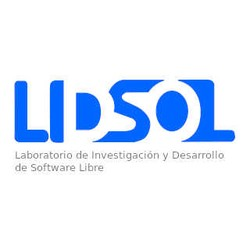# Writing technical content in AcademicImage credit: John Moeses Bauan

Academic is designed to give technical content creators a seamless experience. You can focus on the content and Academic handles the rest.

Highlight your code snippets, take notes on math classes, and draw diagrams from textual representation.

On this page, you'll find some examples of the types of technical content that can be rendered with Academic.

## Examples

### Code

Academic supports a Markdown extension for highlighting code syntax. You can enable this feature by toggling the highlight option in your config/_default/params.toml file.

python
import pandas as pd



renders as

import pandas as pd


### Math

Academic supports a Markdown extension for $\LaTeX$ math. You can enable this feature by toggling the math option in your config/_default/params.toml file and adding markup: mmark to your page front matter.

To render inline or block math, wrap your LaTeX math with $$...$$.

Example math block:

$$\gamma_{n} = \frac{ \left | \left (\mathbf x_{n} - \mathbf x_{n-1} \right )^T \left [\nabla F (\mathbf x_{n}) - \nabla F (\mathbf x_{n-1}) \right ] \right |} {\left \|\nabla F(\mathbf{x}_{n}) - \nabla F(\mathbf{x}_{n-1}) \right \|^2}$$


renders as

$\gamma_{n} = \frac{ \left | \left (\mathbf x_{n} - \mathbf x_{n-1} \right )^T \left [\nabla F (\mathbf x_{n}) - \nabla F (\mathbf x_{n-1}) \right ] \right |}{\left \|\nabla F(\mathbf{x}_{n}) - \nabla F(\mathbf{x}_{n-1}) \right \|^2}$

Example inline math $$\left \|\nabla F(\mathbf{x}_{n}) - \nabla F(\mathbf{x}_{n-1}) \right \|^2$$ renders as $$\left \|\nabla F(\mathbf{x}_{n}) - \nabla F(\mathbf{x}_{n-1}) \right \|^2$$ .

### Diagrams

Academic supports a Markdown extension for diagrams. You can enable this feature by toggling the diagram option in your config/_default/params.toml file or by adding diagram: true to your page front matter.

An example flowchart:

mermaid
graph TD;
A-->B;
A-->C;
B-->D;
C-->D;



renders as

graph TD;
A-->B;
A-->C;
B-->D;
C-->D;


An example sequence diagram:

mermaid
sequenceDiagram
participant Alice
participant Bob
Alice->John: Hello John, how are you?
loop Healthcheck
John->John: Fight against hypochondria
end
Note right of John: Rational thoughts <br/>prevail...
John-->Alice: Great!
John->Bob: How about you?
Bob-->John: Jolly good!



renders as

sequenceDiagram
participant Alice
participant Bob
Alice->John: Hello John, how are you?
loop Healthcheck
John->John: Fight against hypochondria
end
Note right of John: Rational thoughts <br/>prevail...
John-->Alice: Great!
John->Bob: How about you?
Bob-->John: Jolly good!


An example Gantt diagram:

mermaid
gantt
dateFormat  YYYY-MM-DD
section Section
A task           :a1, 2014-01-01, 30d
Another task     :after a1  , 20d
section Another
Task in sec      :2014-01-12  , 12d
another task      : 24d



renders as

gantt
dateFormat  YYYY-MM-DD
section Section
A task           :a1, 2014-01-01, 30d
Another task     :after a1  , 20d
section Another
Task in sec      :2014-01-12  , 12d
another task      : 24d


### Todo lists

You can even write your todo lists in Academic too:

- [x] Write math example
- [x] Write diagram example
- [ ] Do something else


renders as

• Write math example
• Write diagram example
• Do something else

### Tables

Represent your data in tables:

| First Header  | Second Header |
| ------------- | ------------- |
| Content Cell  | Content Cell  |
| Content Cell  | Content Cell  |


renders as

Content Cell Content Cell
Content Cell Content Cell

### Asides

Academic supports a Markdown extension for asides, also referred to as notices or hints. By prefixing a paragraph with A>, it will render as an aside. You can enable this feature by adding markup: mmark to your page front matter, or alternatively using the Alert shortcode.

A> A Markdown aside is useful for displaying notices, hints, or definitions to your readers.


renders as

### Did you find this page helpful? Consider sharing it 🙌##### Laboratorio de Investigación y Desarrollo de Software Libre
###### Laboratorio de investigación

El LIDSOL es un laboratorio formado por voluntarios, alumnos, ex-alumnos y académicos interesados en el desarrollo de tecnologías libres y las discusiones sobre su impacto en la sociedad.